## RD Sharma Solutions for Class 6 Chapter 4 Operations on Whole Numbers Free Online

Exercise 4.1 PAGE: 4.4
1. Fill in the blanks to make each of the following a true statement:
(i) 359 + 476 = 476 + …..
(ii) …. + 1952 = 1952 + 2008
(iii) 90758 + 0 = ….
(iv) 54321 + (489 + 699) = 489 + (54321 + …..)
Solution:
(i) 359 + 476 = 476 + 359 using commutativity
(ii) 2008 + 1952 = 1952 + 2008 using commutativity
(iii) 90758 + 0 = 90758 using the additive identity
(iv) 54321 + (489 + 699) = 489 + (54321 + 699) using associativity
2. Add each of the following and check by reversing the order of addends:
(i) 5628 + 39784
(ii) 923584 + 178
(iii) 15409 + 112
(iv) 2359 + 641
Solution:
(i) We get
5628 + 39784 = 45412
By reversing the order of addends
39784 + 5628 = 45412
(ii) We get
923584 + 178 = 923762
By reversing the order of addends
178 + 923584 = 923762
(iii) We get
15409 + 112 = 15521
By reversing the order of addends
112 + 15409 = 15521
(iv) We get
2359 + 641 = 3000
By reversing the order of addends
641 + 2359 = 3000
3. Determine the sum by suitable rearrangements:
(i) 953 + 407 + 647
(ii) 15409 + 178 + 591 + 322
(iii) 2359 + 10001 + 2641 + 9999
(iv) 1 + 2 + 3 + 4 + 1996 + 1997 + 1998 + 1999
(v) 10 + 11 + 12 + 13 + 14 + 15 + 16 + 17 + 18 + 19 + 20
Solution:
(i) 953 + 407 + 647
We know that
53 + 47 = 100
It can be written as
(953 + 647) + 407 = 1600 + 407
On further calculation
(953 + 647) + 407 = 2007
(ii) 15409 + 178 + 591 + 322
We know that
409 + 91 = 500 and 78 + 22 = 100
It can be written as
(15409 + 591) + (178 + 322) = 16000 + 500
On further calculation
(15409 + 591) + (178 + 322) = 16500
(iii) 2359 + 10001 + 2641 + 9999
We know that
59 + 41 = 100 and 99 + 01 = 100
It can be written as
(2359 + 2641) + (10001 + 9999) = 5000 + 20000
On further calculation
(2359 + 2641) + (10001 + 9999) = 25000
(iv) 1 + 2 + 3 + 4 + 1996 + 1997 + 1998 + 1999
We know that
99 + 1 = 100, 98 + 2 = 100, 97 + 3 = 100 and 96 + 4 = 100
It can be written as
(1 + 1999) + (2 + 1998) + (3 + 1997) + (4 + 1996) = 2000 + 2000 + 2000 + 2000
On further calculation
(1 + 1999) + (2 + 1998) + (3 + 1997) + (4 + 1996) = 8000
(v) 10 + 11 + 12 + 13 + 14 + 15 + 16 + 17 + 18 + 19 + 20
We know that
10 + 20 = 30, 1 + 9 = 10, 2 + 8 = 10, 3 + 7 = 10 and 4 + 6 = 10
It can be written as
(10 + 20) + (11 + 19) + (12 + 18) + (13 + 17) + (14 + 16) = 30 + 30 + 30 + 30 + 30 + 15
On further calculation
(10 + 20) + (11 + 19) + (12 + 18) + (13 + 17) + (14 + 16) = 150 + 15 = 165
4. Which of the following statements are true and which are false:
(i) The sum of two odd numbers is an odd number.
(ii) The sum of two odd numbers is an even number.
(iii) The sum of two even numbers is an even number.
(iv) The sum of two even numbers is an odd number.
(v) The sum of an even number and an odd number is an odd number.
(vi) The sum of an odd number and an even number is an even number.
(vii) Every whole number is a natural number.
(viii) Every natural number is a whole number.
(ix) There is a whole number which when added to a whole number, gives that number.
(x) There is a natural number which when added to a natural number, gives that number.
(xi) Commutativity and associativity are properties of whole numbers.
(xii) Commutativity and associativity are properties of addition of whole numbers.
Solution:
(i) False. We know that, 1 + 3 = 4 where 4 is an even number.
(ii) True. We know that, 5 + 7 = 12 where 12 is an even number.
(iii) True. We know that, 2 + 4 = 6 where 6 is an even number.
(iv) False. We know that, 4 + 6 = 10 where 10 is an even number.
(v) True. We know that, 2 + 1 = 3 where 3 is an odd number.
(vi) False. We know that, 3 + 2 = 5 where 5 is an odd number.
(vii) False. Whole number starts from 0 whereas natural numbers start from 1.
(viii) True. All the natural numbers are also whole number.
(ix) True. We know that, 1 + 0 = 1 where 1 is a whole number.
(x) False. We know that 2 + 1 = 3 which is not that number.
(xi) False. Commutativity and associativity are not properties of whole numbers.
(xii) True. Commutativity and associativity are properties of addition of whole numbers.

Exercise 4.2 PAGE: 4.8
1. A magic square is an array of numbers having the same number of rows and columns and the sum of numbers in each row, column or diagonal being the same. Fill in the blank cells of the following magic squares:
(i)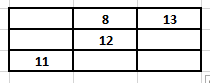(ii)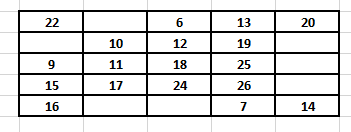Solution:
(i) We know that
Considering diagonal values 13 + 12 + 11 = 36
So we get
No. in the first cell of the first row = 36 – (8 + 13) = 15
No. in the first cell of the second row = 36 – (15 + 11) = 10
No. in the third cell of the second row = 36 – (10 + 12) = 14
No. in the second cell of the third row = 36 – (8 + 12) = 16
No. in the third cell of the third row = 36 – (11 + 16) = 9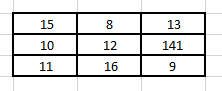(ii) We know that
Considering diagonal values 20 + 19 + 18 + 17 + 16 = 90
So we get
No. in the second cell of the first row = 90 – (22 + 6 + 13 + 20) = 29
No. in the first cell of the second row = 90 – (22 + 9 + 15 + 16) = 28
No. in the fifth cell of the second row = 90 – (28 + 10 + 12 + 19) = 21
No. in the fifth cell of the third row = 90 – (9 + 11 + 18 + 25) = 27
No. in the fifth cell of the fourth row = 90 – (15 + 17 + 24 + 26) = 8
No. in the second cell of the fifth row = 90 – (29 + 10 + 11 + 17) = 23
No. in the third cell of the fifth row = 90 – (6 + 12 + 18 + 24) = 30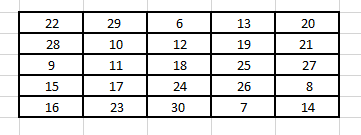2. Perform the following subtractions and check your results by performing corresponding additions:
(i) 57839 – 2983
(ii) 92507 – 10879
(iii) 400000 – 98798
(iv) 5050501 – 969696
(v) 200000 – 97531
(vi) 3030301 – 868686
Solution:
(i) 57839 – 2983
We know that
57839 – 2983 = 54856
54856 + 2983 = 57839
(ii) 92507 – 10879
We know that
92507 – 10879 = 81628
81628 + 10879 = 92507
(iii) 400000 – 98798
We know that
400000 – 98798 = 301202
301202 + 98798 = 400000
(iv) 5050501 – 969696
We know that
5050501 – 969696 = 4080805
4080805 + 969696 = 5050501
(v) 200000 – 97531
We know that
200000 – 97531 = 102469
102469 + 97531 = 200000
(vi) 3030301 – 868686
We know that
3030301 – 868686 = 2161615
2161615 + 868686 = 3030301
3. Replace each * by the correct digit in each of the following:
(i)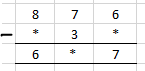(ii)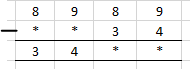(iii)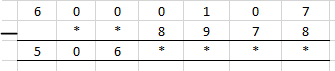(iv)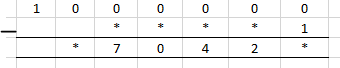(v)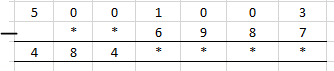(vi)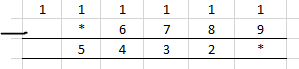Solution:
(i) We know that in the units digit
6 – * = 7 where the value of * is 9 as 1 gets carried from 7 at tens place to 6 at units place
6 at the units place becomes 16 so 16 – 9 = 7
When 7 is reduced by 1 it gives 6 so 6 – 3 = 3
We know that
8 – * = 6 so we get * value as 2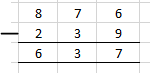(ii) We know that in the units digit
9 – 4 = 5
Tens digit 8 – 3 = 5
So the missing blank can be found by subtracting 3455 from 8989
Difference between them = 3455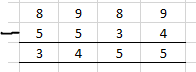(iii) We know that in units digit
17 – 8 = 9
Tens digit = 9 – 7 = 2
So we get
Hundreds place 10 – 9 = 1
Thousands place 9 – 8 = 1
So the addend difference = 5061129
Subtract 5061129 from 6000107 to get addend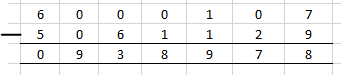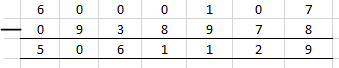(iv) We know that in units digit
10 – 1 = 9
Lakhs place 9 – 0 = 9
So the addend difference = 970429
Subtract 970429 from 1000000 to get the addend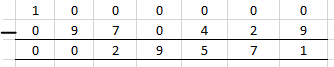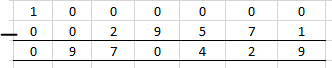(v) We know that in units digit
13 – 7 = 6
Tens digit 9 – 8 = 1
Hundreds place 9 – 9 = 0
Thousands place 10 – 6 = 4
So the addend difference = 4844016
Subtract 4844016 from 5001003 to get the addend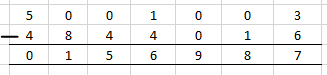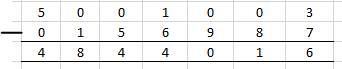(vi) We know that units digit
11 – 9 = 2
So the addend difference = 54322
Subtract 54322 from 111111 to get the addend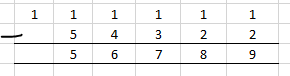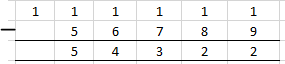4. What is the difference between the largest number of five digits and the smallest number of six digits?
Solution:
99999 is the largest number of five digits
100000 is the largest number of six digits
Difference = 100000 – 99999 = 1
Therefore, 1 is the difference between the largest number of five digits and smallest number of six digits.
5. Find the difference between the largest number of 4 digits and the smallest number of 7 digits.
Solution:
9999 is the largest number of 4 digits
1000000 is the smallest number of 6 digits
Difference = 1000000 – 9999 = 990001
Therefore, 990001 is the difference between the largest number of 4 digits and the smallest number of 7 digits.
6. Rohit deposited Rs 125000 in his savings bank account. Later he withdrew Rs 35425 from it. How much money was left in his account?
Solution:
Money deposited in savings bank account = Rs 125000
Money withdrawn = Rs 35425
So the money which is left out in his account = 125000 – 35425 = Rs 89575
Hence, Rs 89575 is left in his account.
7. The population of a town is 96209. If the number of men is 29642 and that of women is 29167, determine the number of children.
Solution:
Population of a town = 96209
No. of men = 29642
No. of women = 29167
Total number of men and women = 29642 + 29167 = 58809
So the number of children = Population of a town – Total number of men and women
Number of children = 96209 – 58809 = 37400
Hence, there are 37400 children.
8. The digits of 6 and 9 of the number 36490 are interchanged. Find the difference between the original number and the new number.
Solution:
It is given that
Number = 39460
Number after interchanging 6 and 9 = 36490
Difference between them = 39460 – 36490 = 2790
Therefore, the difference between the original number and new number is 2970.
9. The population of a town was 59000. In one year it was increased by 4536 due to new births. However, 9218 persons died or left the town during the year. What was the population at the end of the year?
Solution:
Population of a town = 59000
Population increase = 4536
Population decrease = 9218
So the population at the end of year = 59000 + 4536 – 9218 = 54318
Therefore, the population at the end of the year is 54318.

Exercise 4.3 page: 4.14
1. Fill in the blanks to make each of the following a true statement:
(i) 785 × 0 = …..
(ii) 4567 × 1 = …..
(iii) 475 × 129 = 129 × …..
(iv) ….. × 8975 = 8975 × 1243
(v) 10 × 100 × …. = 10000
(vi) 27 × 18 = 27 × 9 + 27 × ….. + 27 × 5
(vii) 12 × 45 = 12 × 50 – 12 × …..
(viii) 78 × 89 = 78 × 100 – 78 × ….. + 78 × 5
(ix) 66 × 85 = 66 × 90 – 66 × ….. – 66
(x) 49 × 66 + 49 × 34 = 49 × (….. + …..)
Solution:
(i) 785 × 0 = 0
(ii) 4567 × 1 = 4567 based on multiplicative identity
(iii) 475 × 129 = 129 × 475 based on commutativity
(iv) 1243 × 8975 = 8975 × 1243 based on commutativity
(v) 10 × 100 × 10 = 10000
(vi) 27 × 18 = 27 × 9 + 27 × 4 + 27 × 5
(vii) 12 × 45 = 12 × 50 – 12 × 5
(viii) 78 × 89 = 78 × 100 – 78 × 16 + 78 × 5
(ix) 66 × 85 = 66 × 90 – 66 × 4 – 66
(x) 49 × 66 + 49 × 34 = 49 × (66 + 34)
2. Determine each of the following products by suitable rearrangements:
(i) 2 × 1497 × 50
(ii) 4 × 358 × 25
(iii) 495 × 625 × 16
(iv) 625 × 20 × 8 × 50
Solution:
(i) 2 × 1497 × 50
It can be written as
2 × 1497 × 50 = (2 × 50) × 1497
= 100 × 1497
= 149700
(ii) 4 × 358 × 25
It can be written as
4 × 358 × 25 = (4 × 25) × 358
= 100 × 358
= 35800
(iii) 495 × 625 × 16
It can be written as
495 × 625 × 16 = (625 × 16) × 495
= 10000 × 495
= 4950000
(iv) 625 × 20 × 8 × 50
It can be written as
625 × 20 × 8 × 50 = (625 × 8) × (20 × 50)
= 5000 × 1000
= 5000000
3. Using distributivity of multiplication over addition of whole numbers, find each of the following products:
(i) 736 × 103
(ii) 258 × 1008
(iii) 258 × 1008
Solution:
(i) 736 × 103
It can be written as
= 736 × (100 + 3)
By using distributivity of multiplication over addition of whole numbers
= (736 × 100) + (736 × 3)
On further calculation
= 73600 + 2208
We get
= 75808
(ii) 258 × 1008
It can be written as
= 258 × (1000 + 8)
By using distributivity of multiplication over addition of whole numbers
= (258 × 1000) + (258 × 8)
On further calculation
= 258000 + 2064
We get
= 260064
(iii) 258 × 1008
It can be written as
= 258 × (1000 + 8)
By using distributivity of multiplication over addition of whole numbers
= (258 × 1000) + (258 × 8)
On further calculation
= 258000 + 2064
We get
= 260064
4. Find each of the following products:
(i) 736 × 93
(ii) 816 × 745
(iii) 2032 × 613
Solution:
(i) 736 × 93
It can be written as
= 736 × (100 – 7)
By using distributivity of multiplication over subtraction of whole numbers
= (736 × 100) – (736 × 7)
On further calculation
= 73600 – 5152
We get
= 68448
(ii) 816 × 745
It can be written as
= 816 × (750 – 5)
By using distributivity of multiplication over subtraction of whole numbers
= (816 × 750) – (816 × 5)
On further calculation
= 612000 – 4080
We get
= 607920
(iii) 2032 × 613
It can be written as
= 2032 × (600 + 13)
By using distributivity of multiplication over addition of whole numbers
= (2032 × 600) – (2032 × 13)
On further calculation
= 1219200 – 26416
We get
= 1245616
5. Find the values of each of the following using properties:
(i) 493 × 8 + 493 × 2
(ii) 24579 × 93 + 7 × 24579
(iii) 1568 × 184 – 1568 × 84
(iv) 15625 × 15625 – 15625 × 5625
Solution:
(i) 493 × 8 + 493 × 2
It can be written as
= 493 × (8 + 2)
By using distributivity of multiplication over addition of whole numbers
= 493 × 10
On further calculation
= 4930
(ii) 24579 × 93 + 7 × 24579
It can be written as
= 24579 × (93 + 7)
By using distributivity of multiplication over addition of whole numbers
= 24579 × 100
On further calculation
= 2457900
(iii) 1568 × 184 – 1568 × 84
It can be written as
= 1568 × (184 – 84)
By using distributivity of multiplication over subtraction of whole numbers
= 1568 × 100
On further calculation
= 156800
(iv) 15625 × 15625 – 15625 × 5625
It can be written as
= 15625 × (15625 – 5625)
By using distributivity of multiplication over addition subrtaction of whole numbers
= 15625 × 10000
On further calculation
= 156250000
6. Determine the product of:
(i) the greatest number of four digits and the smallest number of three digits.
(ii) the greatest number of five digits and the greatest number of three digits.
Solution:
(i) We know that
Largest four digit number = 9999
Smallest three digit number = 100
Product of both = 9999 × 100 = 999900
Hence, the product of the greatest number of four digits and the smallest number of three digits is 999900.
(ii) We know that
Largest five digit number = 9999
Largest three digit number = 999
Product of both = 9999 × 999
It can be written as
= 9999 × (1000 – 1)
By using distributivity of multiplication over addition subrtaction of whole numbers
= (9999 × 1000) – (9999 × 1)
On further calculation
= 9999000 – 9999
We get
= 9989001
7. In each of the following, fill in the blanks, so that the statement is true:
(i) (500 + 7) (300 – 1) = 299 × …..
(ii) 888 + 777 + 555 = 111 × …..
(iii) 75 × 425 = (70 + 5) (….. + 85)
(iv) 89 × (100 – 2) = 98 × (100 – …..)
(v) (15 + 5) (15 – 5) = 225 – …..
(vi) 9 × (10000 + …..) = 98766
Solution:
(i) By considering LHS
(500 + 7) (300 – 1)
We get
= 507 × 299
By using commutativity
= 299 × 507
(ii) By considering LHS
888 + 777 + 555
We get
= 111 (8 + 7 + 5)
By using distributivity
= 111 × 20
(iii) By considering LHS
75 × 425
We get
= (70 + 5) × 425
It can be written as
= (70 + 5) (340 + 85)
(iv) By considering LHS
89 × (100 – 2)
We get
= 89 × 98
It can be written as
= 98 × 89
By using commutativity
= 98 × (100 – 11)
(v) By considering LHS
(15 + 5) (15 – 5)
We get
= 20 × 10
On further calculation
= 200
It can be written as
= 225 – 25
(vi) By considering LHS
9 × (10000 + 974) = 98766
8. A dealer purchased 125 colour television sets. If the cost of each set is Rs 19820, determine the cost of all sets together.
Solution:
It is given that
Cost of each television set = Rs 19820
So we get
Cost of 125 television sets = 19820 × 125
It can be written as
= 19820 × (100 + 25)
By using distributivity of multiplication over addition subrtaction of whole numbers
= (19820 × 100) + (19820 × 25)
On further calculation
= 1982000 + 495500
So we get
= Rs 2477500
9. The annual fee charged from a student of class VI in a school is Rs 8880. If there are, in all, 235 students in class VI, find the total collection.
Solution:
Annual fee per student = Rs 8800
So we get
Annual fee charged for 235 students = 8800 × 235 = 2086800
Therefore, the total collection is Rs 2086800.
10. A group housing society constructed 350 flats. If the cost of construction for each flat is Rs 993570, what is the total cost of construction of all the flats.
Solution:
Cost of construction for each flat = Rs 993570
Number of flats constructed = 350
So we get
Cost of construction of 350 flats = 993570 × 350 = Rs 347749500
Therefore, the total cost of construction of all the flats is Rs 347749500.
11. The product of two whole numbers is zero. What do you conclude?
Solution:
The product of two whole numbers is zero, which means that at least one number or both of them are zero.
12. What are the whole numbers which when multiplied with itself gives the same number?
Solution:
Two numbers when multiplied with itself gives the same number.
For example: 0 × 0 = 0 and 1 × 1 = 1
13. In a large housing complex, there are 15 small buildings and 22 large building. Each of the large buildings has 10 floors with 2 apartments on each floor. Each of the small buildings has 12 floors with 3 apartments on each floor. How many apartments are there in all.
Solution:
It is given that
No. of large buildings = 22
No. of small buildings = 15
No. of floors in 1 large building = 10
No. of apartments on 1 floor = 2
So total apartment in 1 large building = 10 × 2 = 20
The same way
No. of apartments in 1 small building = 12 × 3 = 36
So the total apartment in entire housing complex = (22 × 20) + (15 × 36) = 440 + 540 = 980
Therefore, there are 980 apartments in all.

Exercise 4.4 page: 4.19
1. Does there exist a whole number a such that a ÷ a = a?
Solution:
Yes. There exists a whole number ‘a’ such that a ÷ a = a.
We know that the whole number is 1 where 1 ÷ 1 = 1.
2. Find the value of:
(i) 23457 ÷ 1
(ii) 0 ÷ 97
(iii) 476 + (840 ÷ 84)
(iv) 964 – (425 ÷ 425)
(v) (2758 ÷ 2758) – (2758 ÷ 2758)
(vi) 72450 ÷ (583 – 58)
Solution:
(i) 23457 ÷ 1
By division
23457 ÷ 1 = 23457
(ii) 0 ÷ 97
By division
0 ÷ 97 = 0
(iii) 476 + (840 ÷ 84)
On further calculation
476 + (840 ÷ 84) = 476 + 10
= 486
(iv) 964 – (425 ÷ 425)
On further calculation
964 – (425 ÷ 425) = 964 – 1
= 963
(v) (2758 ÷ 2758) – (2758 ÷ 2758)
On further calculation
(2758 ÷ 2758) – (2758 ÷ 2758) = 1 – 1
= 0
(vi) 72450 ÷ (583 – 58)
On further calculation
72450 ÷ (583 – 58) = 72450 ÷ 525
= 138
3. Which of the following statements are true:
(i) 10 ÷ (5 × 2) = (10 ÷ 5) × (10 ÷ 2)
(ii) (35 – 14) ÷ 7 = 35 ÷ 7 – 14 ÷ 7
(iii) 35 – 14 ÷ 7 = 35 ÷ 7 – 14 ÷ 7
(iv) (20 – 5) ÷ 5 = 20 ÷ 5 – 5
(v) 12 × (14 ÷ 7) = (12 × 14) ÷ (12 × 7)
(vi) (20 ÷ 5) ÷ 2 = (20 ÷ 2) ÷ 5
Solution:
(i) False.
We know that
LHS = 10 ÷ (5 × 2)
So we get
= 10 ÷ 10
= 1
RHS = (10 ÷ 5) × (10 ÷ 2)
So we get
= 2 × 5
= 10
(ii) True.
We know that
LHS = (35 – 14) ÷ 7
So we get
= 21 ÷ 7
= 3
RHS = 35 ÷ 7 – 14 ÷ 7
So we get
= 5 – 2
= 3
(iii) False.
We know that
LHS = 35 – 14 ÷ 7
So we get
= 35 – 2
= 33
RHS = 35 ÷ 7 – 14 ÷ 7
So we get
= 5 – 2
= 3
(iv) False.
We know that
LHS = (20 – 5) ÷ 5
So we get
= 15 ÷ 5
= 3
RHS = 20 ÷ 5 – 5
So we get
= 4 – 5
= – 1
(v) False.
We know that
LHS = 12 × (14 ÷ 7)
So we get
= 12 × 2
= 24
RHS = (12 × 14) ÷ (12 × 7)
So we get
= 168 ÷ 84
= 2
(vi) True.
We know that
LHS = (20 ÷ 5) ÷ 2
So we get
= 4 ÷ 2
= 2
RHS = (20 ÷ 2) ÷ 5
So we get
= 10 ÷ 5
= 2
4. Divide and check the quotient and remainder:
(i) 7772 ÷ 58
(ii) 6906 ÷ 35
(iii) 16135 ÷ 875
(iv) 16025 ÷ 1000
Solution:
(i) 7772 ÷ 58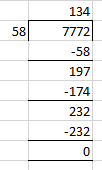So we get 7772 ÷ 58 = 134
By verifying
We know that
Dividend = Divisor × Quotient + Remainder
By substituting values
7772 = 58 × 134 + 0
So we get
7772 = 7772
LHS = RHS
(ii) 6906 ÷ 35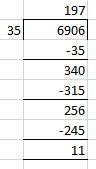So we get quotient = 197 and remainder = 11
By verifying
We know that
Dividend = Divisor × Quotient + Remainder
By substituting values
6906 = 35 × 197 + 11
On further calculation
6906 = 6895 + 11
We get
6906 = 6906
LHS = RHS
(iii) 16135 ÷ 875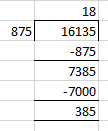So we get quotient = 18 and remainder = 385
By verifying
We know that
Dividend = Divisor × Quotient + Remainder
By substituting values
16135 = 875 × 18 + 385
On further calculation
16135 = 15750 + 385
We get
16135 = 16135
LHS = RHS
(iv) 16025 ÷ 1000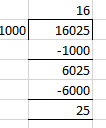So we get quotient = 16 and remainder = 25
By verifying
We know that
Dividend = Divisor × Quotient + Remainder
By substituting values
16025 = 1000 × 16 + 25
On further calculation
16025 = 16000 + 25
We get
16025 = 16025
LHS = RHS
5. Find a number which when divided by 35 gives the quotient 20 and remainder 18.
Solution:
We know that
Dividend = Divisor × Quotient + Remainder
By substituting values
Dividend = 35 × 20 + 18
On further calculation
Dividend = 700 + 18
So we get
Dividend = 718
6. Find the number which when divided by 58 gives a quotient 40 and remainder 31.
Solution:
We know that
Dividend = Divisor × Quotient + Remainder
By substituting values
Dividend = 58 × 40 + 31
On further calculation
Dividend = 2320 + 31
So we get
Dividend = 2351
7. The product of two numbers is 504347. If one of the numbers is 1591, find the other.
Solution:
The product of two numbers = 504347
One of the numbers = 1591
Consider A as the number
A × 1591 = 504347
So by division
A = 317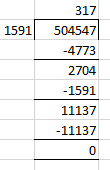8. On dividing 59761 by a certain number, the quotient is 189 and the remainder is 37. Find the divisor.
Solution:
It is given that
Dividend = 59761
Quotient = 189
Remainder = 37
Consider Divisor = A
We know that
Dividend = Divisor × Quotient + Remainder
By substituting values
59761 = A × 189 + 37
On further calculation
59761 – 37 = A × 189
So we get
59724 = A × 189
By division
A = 316
9. On dividing 55390 by 299, the remainder is 75. Find the quotient.
Solution:
It is given that
Dividend = 55390
Quotient = 299
Remainder = 75
Consider Divisor = A
We know that
Dividend = Divisor × Quotient + Remainder
By substituting values
55390 = A × 299 + 75
On further calculation
55390 – 75 = A × 299
So we get
55315 = A × 299
By division
A = 185

Exercise 4.5 page: 4.23
1. Without drawing a diagram, find
(i) 10th square number
(ii) 6th triangular number
Solution:
(i) 10th square number
The square number can be remembered using the following rule
Nth square number = n × n
So the 10th square number = 10 × 10 = 100
(ii) 6th triangular number
The triangular number can be remembered using the following rule
Nth triangular number = n × (n + 1)/ 2
So the 6th triangular number = 6 × (6 + 1)/ 2 = 21
2. (i) Can a rectangular number also be a square number?
(ii) Can a triangular number also be a square number?
Solution:
(i) Yes. A rectangular number can also be a square number.
Example – 16 is a rectangular number which can also be a square number.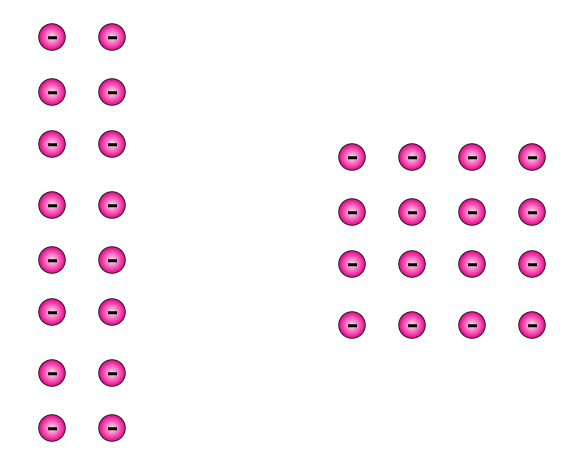(ii) Yes. A triangular number can also be a square number.
Example – 1 is a triangular number which can also be a square number.
3. Write the first four products of two numbers with difference 4 starting from in the following order:
1, 2, 3, 4, 5, 6, ………
Identify the pattern in the products and write the next three products.
Solution:
We know that
1 × 5 = 5
2 × 6 = 12
3 × 7 = 21
4 × 8 = 32
So the first four products of two numbers with difference 4
5 – 1 = 4
6 – 2 = 4
7 – 3 = 4
8 – 4 = 4
4. Observe the pattern in the following and fill in the blanks:
9 × 9 + 7 = 88
98 × 9 + 6 = 888
987 × 9 + 5 = 8888
9876 × 9 + 4 = ………
98765 × 9 + 3 = ………
987654 × 9 + 2 = ……….
9876543 × 9 + 1 = ……….
Solution:
9 × 9 + 7 = 88
98 × 9 + 6 = 888
987 × 9 + 5 = 8888
9876 × 9 + 4 = 88888
98765 × 9 + 3 = 888888
987654 × 9 + 2 = 8888888
9876543 × 9 + 1 = 88888888
5. Observe the following pattern and extend it to three more steps:
6 × 2 – 5 = 7
7 × 3 – 12 = 9
8 × 4 – 21 = 11
9 × 5 – 32 = 13
….. × ……. – ….. = …….
….. × ……. – ….. = …….
….. × ……. – ….. = …….
Solution:
6 × 2 – 5 = 7
7 × 3 – 12 = 9
8 × 4 – 21 = 11
9 × 5 – 32 = 13
10 × 6 – 45 = 15
11 × 7 – 60 = 17
12 × 8 – 77 = 19
6. Study the following pattern:
1 + 3 = 2 × 2
1 + 3 + 5 = 3 × 3
1 + 3 + 5 + 7 = 4 × 4
1 + 3 + 5 + 7 + 9 = 5 × 5
By observing the above pattern, find
(i) 1 + 3 + 5 + 7 + 9 + 11
(ii) 1 + 3 + 5 + 7 + 9 + 11 + 13 + 15
(iii) 21 + 23 + 25 + ….. + 51
Solution:
(i) 1 + 3 + 5 + 7 + 9 + 11
By using the pattern
1 + 3 + 5 + 7 + 9 + 11 = 6 × 6
= 36
(ii) 1 + 3 + 5 + 7 + 9 + 11 + 13 + 15
By using the pattern
1 + 3 + 5 + 7 + 9 + 11 + 13 + 15 = 8 × 8
= 64
(iii) 21 + 23 + 25 + ….. + 51
We know that
21 + 23 + 25 + ….. + 51 can be written as (1 + 3 + 5 + 7 + ….. + 49 + 51) – (1 + 3 + 5 + …… + 17 + 19)
By using the pattern
(1 + 3 + 5 + 7 + ….. + 49 + 51) = 26 × 26 = 676
(1 + 3 + 5 + …… + 17 + 19) = 10 × 10 = 100
So we get
21 + 23 + 25 + ….. + 51 = 676 – 100 = 576
7. Study the following pattern:
1 × 1 + 2 × 2 = (2 × 3 × 5)/ 6
1 × 1 + 2 × 2 + 3 × 3 = (3 × 4 × 7) / 6
1 × 1 + 2 × 2 + 3 × 3 + 4 × 4 = (4 × 5 × 9)/ 6
By observing the above pattern, write next two steps.
Solution:
By using the pattern
1 × 1 + 2 × 2 + 3 × 3 + 4 × 4 + 5 × 5
On further calculation
= 5 × 6 × 116
So we get
= 55
By using the pattern
1 × 1 + 2 × 2 + 3 × 3 + 4 × 4 + 5 × 5 + 6 × 6
On further calculation
= 6 × 7 × 136
So we get
= 91
8. Study the following pattern:
1 = (1 × 2)/ 2
1 + 2 = (2 × 3)/ 2
1 + 2 + 3 = (3 × 4)/ 2
1 + 2 + 3 + 4 = (4 × 5)/ 2
By observing the above pattern, find
(i) 1 + 2 + 3 + 4 + 5 + 6 + 7 + 8 + 9 + 10
(ii) 50 + 51 + 52 + ……. + 100
(iii) 2 + 4 + 6 + 8 + 10 + …….. + 100
Solution:
(i) 1 + 2 + 3 + 4 + 5 + 6 + 7 + 8 + 9 + 10
We get
= 10 × 112
On further calculation
= 55
(ii) 50 + 51 + 52 + ……. + 100
We can write it as
(1 + 2 + 3 + …… + 99 + 100) – (1 + 2 + 3 + 4 + ….. + 47 + 49)
So we get
(1 + 2 + 3 + …… + 99 + 100) = 100 × 1012
(1 + 2 + 3 + 4 + ….. + 47 + 49) = 49 × 502
By substituting the values
50 + 51 + 52 + ……. + 100 = 100 × 1012 + 49 × 502
On further calculation
= 5050 – 1225
We get
= 3825
(iii) 2 + 4 + 6 + 8 + 10 + …….. + 100
We can write it as
2 (1 + 2 + 3 + 4 + …… + 49 + 50)
So we get
= 2 (50 × 512)
On further calculation
= 2 (1275)
We get
= 2550
Objective Type Questions PAGE: 4.24
Mark the correct alternative in each of the following:
1. Which one of the following is the smallest whole number?
(a) 1                     (b) 2                         (c) 0                                     (d) None of these
Solution:
The option (c) is correct answer.
We know that the set of whole numbers is {0, 1, 2, 3, 4 …}.
Hence, the smallest whole number is 0.
2. Which one of the following is the smallest even whole number?
(a) 0                               (b) 1                                   (c) 2                                      (d) None of these
Solution:
The option (c) is correct answer.
We know that the natural numbers along with 0 form the collection of whole numbers.
Hence, the numbers 0, 1, 2, 3, 4 … form the collection of whole numbers.
So the number which is divisible by 2 is an even number and 2 is the smallest even number.
3. Which one of the following is the smallest odd whole number?
(a) 0                               (b) 1                                   (c) 3                                      (d) 5
Solution:
The option (b) is correct answer.
We know that the natural numbers along with 0 form the collection of whole numbers.
Hence, the numbers 0, 1, 2, 3, 4 … form the collection of whole numbers.
So the natural number which is not divisible by 2 is called an odd whole number and 1 is the smallest odd whole number.
4. How many whole numbers are between 437 and 487?
(a) 50                               (b) 49                                   (c) 51                                      (d) None of these
Solution:
The option (b) is correct answer.
We know that the whole numbers between 437 and 487 are 438, 439, 440, 441, …, 484, 485 and 486.

In order to find the required number of whole numbers subtract 437 from 487 and then subtract again 1.

Hence, there are (487 − 437) − 1 whole numbers lying between 437 and 487.

So we get (487 − 437) − 1 = 50 − 1 = 49
5. The product of the successor of 999 and the predecessor of 1001 is
(a) one lakh                            (b) one billion                               (c) one million                                (d) one crore
Solution:
The option (c) is correct answer.
We know that the successor of 999 = 999 + 1 = 1000

So the predecessor of 1001 = 1001 − 1 = 1000

It can be written as
Product of them = (Successor of 999) × (Predecessor of 1001)
By substituting the values
Product of them = 1000 × 1000 = 1000000 = one million
6. Which one of the following whole numbers does not have a predecessor?
(a) 1                            (b) 0                              (c) 2                               (d) None of these
Solution:
The option (b) is correct answer.
We know that the numbers 0, 1, 2, 3, 4 … form the collection of whole numbers.
Hence, the smallest whole number is 0 which does not have a predecessor.
7. The number of whole numbers between the smallest whole number and the greatest 2-digit number is
(a) 101                            (b) 100                              (c) 99                               (d) 98
Solution:
The option (d) is correct answer.
We know that the smallest whole number = 0

So the greatest 2 digit whole number = 99

Whole numbers which lie between 0 and 99 are 1, 2, 3, 4,…, 97, 98.

In order to find the number of whole numbers between 0 and 99, first subtract 1 from the difference of 0 and 99.

So the number of whole numbers between 0 and 99 = (99 − 0) − 1 = 99 − 1 = 98
8. If n is a whole number such that n + n = n, then n =?
(a) 1                            (b) 2                              (c) 3                               (d) None of these
Solution:
The option (d) is correct answer.
We know that 0 + 0 = 0, 1 + 1 = 2, 2 + 2 = 4….

Hence, the statement n + n = n is true only when n = 0.
9. The predecessor of the smallest 3-digit number is
(a) 999                            (b) 99                              (c) 100                               (d) 101
Solution:
The option (b) is correct answer.
We know that the smallest 3 digit number = 100

So the predecessor of 3 digit number = 100 − 1 = 99
10. The least number of 4-digits which is exactly divisible by 9 is
(a) 1008                            (b) 1009                              (c) 1026                               (d) 1018
Solution:
The option (a) is correct answer.
We know that the least 4-digit number = 1000

Hence, the least 4-digits which is exactly divisible by 9 is 1000 + (9 − 1) = 1008
11. The number which when divided by 53 gives 8 as quotient and 5 as remainder is
(a) 424                 (b) 419                       (c) 429                              (d) None of these
Solution:
The option (c) is correct answer.
It is given that
Divisor = 53, Quotient = 8 and Remainder = 5.

By using the relation we get
Dividend = Divisor × Quotient + Remainder

By substituting the values
Dividend = 53 × 8 + 5 = 424 + 5 =429

Hence, the required number is 429.
12. The whole number n satisfying n + 35 = 101 is
(a) 65                 (b) 67                       (c) 64                              (d) 66
Solution:
The option (d) is correct answer.
It is given that
n + 35 = 101

By adding − 35 on both sides

n + 35 + (− 35) = 101 + (− 35)
On further calculation
n + 0 = 66

So we get
n = 66
13. The 4 × 378 × 25 is
(a) 37800                 (b) 3780                       (c) 9450                              (d) 30078
Solution:
The option (a) is correct answer.
We can write it as
4 × 378 × 25 = 4 × 25 × 378

On further calculation
4 × 378 × 25 = 100 × 378 = 37800
14. The value of 1735 × 1232 − 1735 × 232 is
(a) 17350                 (b) 173500                       (c) 1735000                              (d) 173505
Solution:
The option (c) is correct answer.
By using the distributive law of multiplication over subtraction

1735 × 1232 − 1735 × 232 = 1735(1232 − 232)

On further calculation
1735 × 1232 − 1735 × 232 = 1735 × 1000 = 1735000
15. The value of 47 × 99 is
(a) 4635                 (b) 4653                       (c) 4563                              (d) 6453
Solution:
The option (b) is correct answer.
It can be written as
99 = 100 − 1

So we get
47 × 99 = 47 × (100 − 1)

On further calculation
47 × 99 = 47× 100 – 47 = 4700 – 47 = 4653

Hence, the value of 47 × 99 is 4653.
Courtesy : CBSE